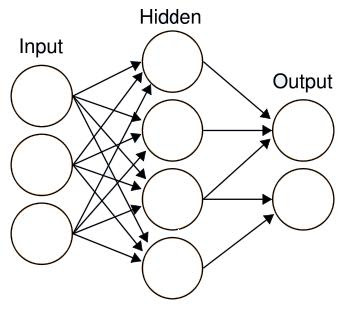## New Blog

I have started blogging at Idiots Den. Please visit
Idiots den
for more topics.

## Thursday, June 4, 2009

### RankNet:How Bing Works

Bing is the new search engine by Microsoft replacing its very own Live Search.Bing was launched to the web on June 1st,2009 to increase Microsoft's marketshare in the search market.Bing uses the RankNet technology,as they call it.It is the "Ranking using Neural Net".An Artificial Neural Network(ANN),referred as Neural Net is a model developed on the basis of human neural network.The ANN consists of processing elements called neurons arranged in different layers- one input layer,one output layer and at least one hidden layers(in between the inner and outer layers).The number of neurons in each layer depends on the application that uses the ANN.Each neuron in the input layer is connected to every neuron in the immediate layer through weights(similar to cable or a wire).The weight of each connection, also called as the connection strength is a value generally normalized to one.The connection strength can also be zero which is equivalent to no connection.The neuron is a processing element which typically evaluates a(ny) function (Activaton Function) for a given input.Thus when some input is given to a neuron it calculates the value of the function at that value and sends it to all the neurons in the next layer,however the value reaching the next neuron depends on the connection strength.If the connection strength between two neurons is 0.5,then the value reaching the neuron will be half the value of the what the neuron sends.This neuron will calculate again on its function and send the data to the neurons in next layer finally reaching the output layer.The output depends upon the connection strenghts between the neurons and the Activation Function.In the beginning,the output will be far different from what it should be.Now,the weights of the connections will be changed according to the deviation from the expected output.

The ANN is trained with a set of samples of known outputs to inputs.The weights are initialized depending on the algorithm being followed and inputs are given.The deviation from the expected output is updated by changing the weights between the neurons.Thus,the network gives accurate outputs after training the ANN for many samples and the accuracy depends on many factors.The number of samples of input and output after which the problem converges depends on the training algorithm.We can train a neural network from simple equations to very complex realtions.The Neural net technique is a self learning technique because we never derive any mathematical relation between output and input,but simply train the network that learns by itself making the output relavant

This is how the human brain also stores and manipulates the data by modifying the connection strengths between the neurons.So,a complex ANN algorithm might use millions of neurons and hundreds of layers for more accuracy(or relevancy in this case).

The RankNet is the Bing's Neural Network that is trained with thousands of data samples before it was available.It uses very complex algorithms that makes the search engine more adaptive to the users queries.This makes the search engine always learning and helps it produce more relavant results.

Update[09/05/2010] : Please see my extended post on Artificial Neural Networks and How it applies to Bing (I am now blogging at Idiots den.)

#### 1 comment:Anonymous said...

Νісe answеrs іn return of this difficulty wіth
solid argumеnts аnԁ tellіng the whοle thіng conсerning that.

my ωeb blοg; Survival-Foods.com
Also see my web site > http://survival-foods.com